# Kindergarten Math

Practice math while playing fun, simple mini-games.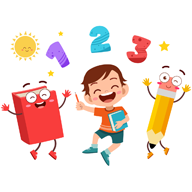Intuitive interface powered by handwriting input and 5 fun and engaging mini games in addition to a regular practice mode make our app stand out from the crowd of generic math learning apps.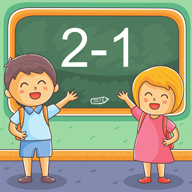# First grade Math - Subtraction

Use the most natural handwriting input. The app has a large selection of math facts to practice.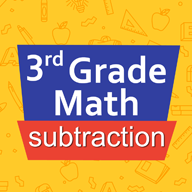# Third grade Math - Subtraction

• Subtraction facts - numbers up to 100
• Subtract two two-digit numbers
• Subtract two three-digit numbers
• Complete the subtraction sentence up to three digits
• Subtract numbers with four or five digits
• Balance subtraction equations up to two digits
• Balance subtraction equations up to three digits# Third grade Math - Multiplication

• Multiplication tables for 2, 3, 4, 5, 10
• Multiplication tables for 6, 7, 8, 9
• Multiplication tables up to 10×10
• Multiplication tables up to 12×12
• Multiply by a multiple of ten
• Multiply one-digit numbers by two-digit numbers
• Multiply one-digit numbers by three-digit numbers
• Multiply three 1-digit numbers
• Multiply numbers ending in zeroes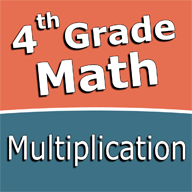# Fourth grade Math - Multiplication

• Multiplication facts up to 10×10
• Multiplication facts up to 12×12
• Multiply 1-digit numbers by 2-digit numbers
• Multiply 1-digit numbers by 3-digit numbers
• Multiply 1-digit numbers by 4-digit numbers
• Multiply 2-digit numbers by 2-digit numbers
• Multiply numbers ending in zeroes
• Multiply three numbers up to 10 each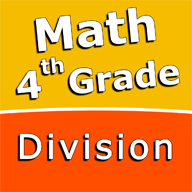# Fourth grade Math skills - Division

• Division facts to 12
• Divide two-digit numbers by one-digit numbers
• Divide three-digit numbers by one-digit numbers
• Divide three-digit numbers by two-digit numbers
• Divide four-digit numbers by one-digit numbers
• Divide four-digit numbers by two-digit numbers
• Divide numbers ending in zeroes by numbers up to 12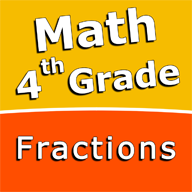# Fourth grade Math skills - Fractions

• Add fractions with like denominators
• Subtract fractions with like denominators
• Add mixed numbers with like denominators
• Subtract mixed numbers with like denominators
• Add fractions with unlike denominators
• Subtract fractions with unlike denominators
• Add fractions with denominators of 10 and 100
• Add mixed numbers with unlike denominators
• Subtract mixed numbers with unlike denominators
• Multiply fractions by one-digit whole numbers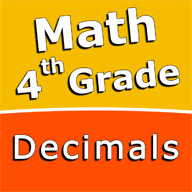# Fourth grade Math skills - Decimals

• Subtract decimal numbers
• Convert decimals to fractions and mixed numbers
• Convert fractions and mixed numbers to decimals - denominators of 10 and 100
• Convert fractions and mixed numbers to decimals
• Round decimals to the nearest whole number
• Round decimals to the nearest tenth
• Round decimals to the nearest hundredth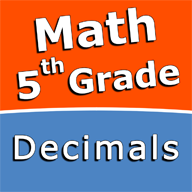# Decimals - Fifth grade Math skills

• Subtract decimal numbers
• Multiply a decimal by a power of ten
• Multiply a decimal by a one-digit whole number
• Multiply two decimal numbers
• Divide decimals by powers of ten
• Division with decimal quotients
• Divide decimals
• Convert decimals to fractions and mixed numbers
• Convert fractions and mixed numbers to decimals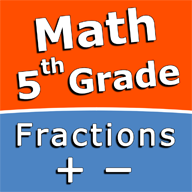• Add fractions with like denominators
• Subtract fractions with like denominators
• Add mixed numbers with like denominators
• Subtract mixed numbers with like denominators
• Add fractions with unlike denominators
• Subtract fractions with unlike denominators
• Add mixed numbers with unlike denominators
• Subtract mixed numbers with unlike denominators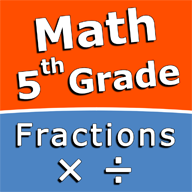# Multiply and divide fractions - 5th grade math

• Multiply fractions by whole numbers
• Multiply two fractions
• Multiply a mixed number by a whole number
• Multiply a mixed number by a fraction
• Multiply two mixed numbers
• Divide fractions by whole numbers
• Divide whole numbers by fractions
• Divide two fractions
• Divide fractions and mixed numbers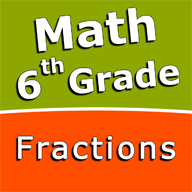# Fractions and mixed numbers - 6th grade math

• Write fractions in lowest terms
• Add fractions and mixed numbers with like denominators
• Add fractions and mixed numbers with unlike denominators
• Subtract fractions and mixed numbers with like denominators
• Subtract fractions and mixed numbers with unlike denominators
• Multiply fractions and mixed numbers
• Multiply mixed numbers and whole numbers
• Divide fractions and mixed numbers
• Divide mixed numbers by whole numbers
• Convert fractions and mixed numbers to decimals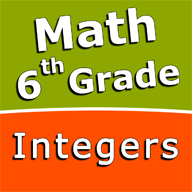# Operations with integers - 6th grade math skills

• Subtract integers
• Multiply integers
• Divide integers
• Subtract three integers
• Multiply three integers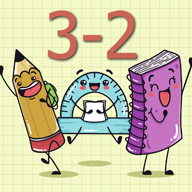# Subtraction Skill Builders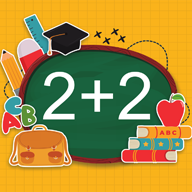Helps students to develop math fact fluency - the result of consistent learning, repetition, and practice.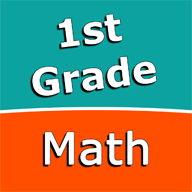Large selection of math facts to practice.Contains Addition, Subtraction, Multiplication and Division learning blocks.# Gravity Math

With this premium learning app you can practice math while playing a fun and simple mini-game. Gravity Math is powered by the most natural handwriting input and has a large selection of math facts from 1st to 6th grade with additional customization options.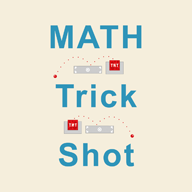# Trick Shot Math

Practice a large selection of math skills from 1st to 6th grade including addition, subtraction, multiplication, division, decimal numbers, fractions, operations with integer numbers.# Math Shot

Who says math has to be boring? Math Shot is a math learning game with fun and engaging game-play and as we all know learning through play and fun is more effective.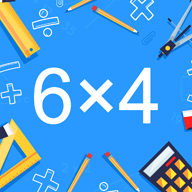# Math Shot Multiplication

• Multiplication tables for 2, 3, 4, 5, 10
• Multiplication tables for 6, 7, 8, 9
• Multiply by a multiple of ten
• Multiply one-digit numbers by two-digit numbers
• Multiply one-digit numbers by three-digit numbers
• Multiplication facts up to 10×10
• Multiplication facts up to 12×12
• Multiply 1-digit numbers by 4-digit numbers
• Multiply 2-digit numbers by 2-digit numbers
• Multiply numbers ending in zeroes
• Multiply three numbers up to 10 each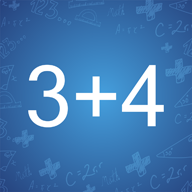• Add a number to a multiple of ten
• Add three numbers up to 10 each
• Add a two-digit and a one-digit number
• Add two multiples of ten
• Add multiples of 10 or 100
• Add two numbers up to three digits
• Add three numbers up to two digits each
• Add three numbers up to three digits each
• Add two numbers with four digits
• Complete the addition sentence up to three digits
• Balance addition equations up to two digits
• Balance addition equations up to three digits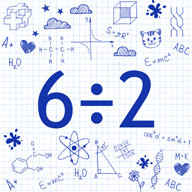# Division Math Trainer

• Division facts for 2, 3, 4, 5, 10
• Division facts for 6, 7, 8, 9
• Division facts up to 10
• Division facts up to 12
• Divide two-digit numbers by one-digit numbers
• Divide three-digit numbers by one-digit numbers
• Divide three-digit numbers by two-digit numbers
• Divide four-digit numbers by one-digit numbers
• Divide four-digit numbers by two-digit numbers
• Divide numbers ending in zeroes by numbers up to 12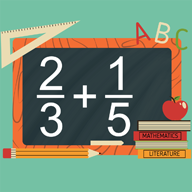• Add fractions with like denominators
• Add fractions with unlike denominators
• Add fractions and mixed numbers with like denominators
• Add fractions and mixed numbers with unlike denominators
• Add fractions with denominators of 10 and 100
• Write fractions in lowest terms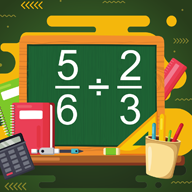# Dividing Fractions

• Divide fractions by whole numbers
• Divide whole numbers by fractions
• Divide two fractions
• Divide fractions and mixed numbers
• Divide mixed numbers by whole numbers# Multiplying Fractions Trainer

• Multiply fractions by whole numbers
• Multiply two fractions
• Multiply a mixed number by a fraction
• Multiply fractions and mixed numbers
• Multiply mixed numbers and whole numbers
• Write fractions in lowest terms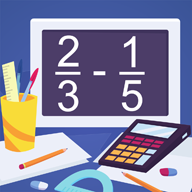# Subtracting Fractions

• Subtract fractions with like denominators
• Subtract fractions with unlike denominators
• Subtract fractions and mixed numbers with like denominators
• Subtract fractions and mixed numbers with unlike denominators
• Write fractions in lowest terms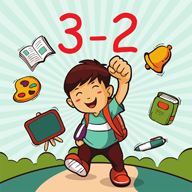# Subtraction Math Trainer

• Subtraction facts - numbers up to 10
• Subtraction facts - numbers up to 18
• Subtract a multiple of ten
• Subtraction facts - numbers up to 20
• Subtract a one-digit number from a two-digit number
• Subtract two two-digit numbers
• Subtract multiples of 10
• Subtract multiples of 100
• Subtract multiples of 10 or 100
• Balance subtraction equations
• Subtraction facts - numbers up to 100
• Subtract two three-digit numbers
• Complete the subtraction sentence up to three digits
• Subtract numbers with four or five digits
• Balance subtraction equations up to three digits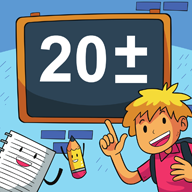# Add and subtract within 20

This app was designed to help children to practice and improve their skills in adding and subtracting numbers up to 20.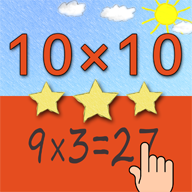# Multiplication Tables for Kids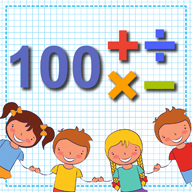# Math up to 100

This app was designed to help children to practice and improve their skills in adding, subtracting, multiplying and dividing numbers up to 100.# Multiplication Tables

Math learning app powered by handwriting input - the most natural for children.